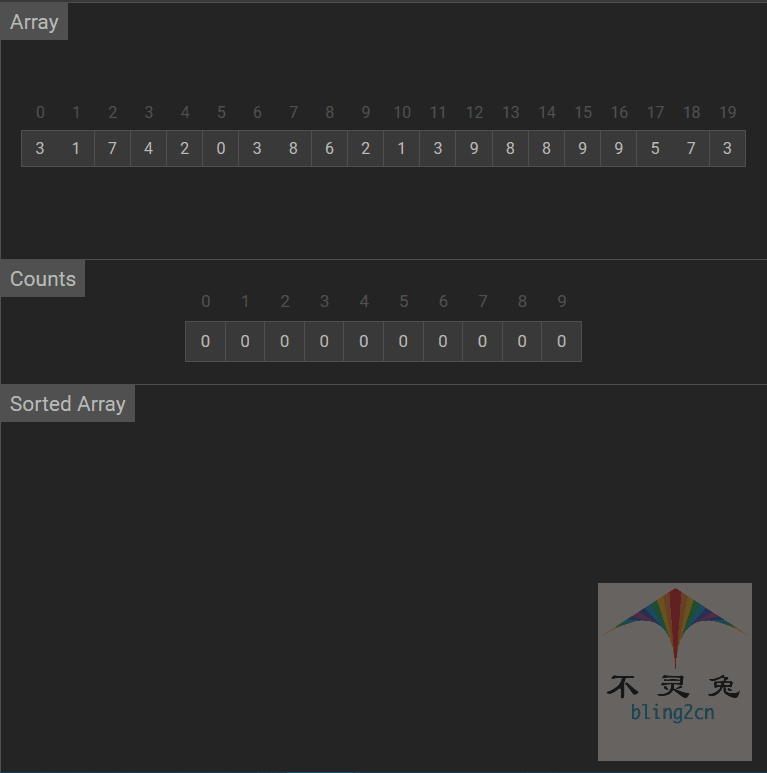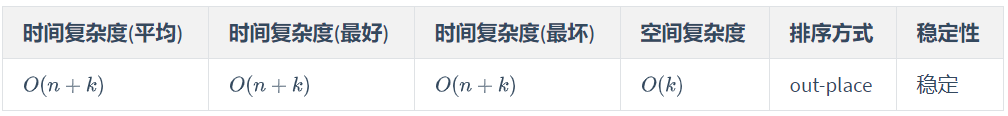# python实现·十大排序算法之计数排序(Counting Sort)## 算法实现步骤

1. 根据待排序集合中最大元素和最小元素的差值范围，申请额外空间；

2. 遍历待排序集合，将每一个元素出现的次数记录到元素值对应的额外空间内；

3. 对额外空间内数据进行计算，得出每一个元素的正确位置；

4. 将待排序集合每一个元素移动到计算得出的正确位置上。

## Python 代码实现

# counting_sort 代码实现from typing import List    def counting_sort(arr:List[int]):	max=min=0	for i in arr:		if i < min:			min = i		if i > max:			max = i 	count =  * (max - min +1)	for j in range(max-min+1):		count[j]=0	for index in arr:		count[index-min]+=1	index=0	for a in range(max-min+1):		for c in range(count[a]):			arr[index]=a+min			index+=1

# 测试数据if __name__ == '__main__':    import random    random.seed(54)    arr = [random.randint(0,100) for _ in range(10)]    print("原始数据：", arr)    counting_sort(arr)    print("计数排序结果", arr)

# 输出结果原始数据： [17, 56, 71, 38, 61, 62, 48, 28, 57, 42]计数排序结果 [17, 28, 38, 42, 48, 56, 57, 61, 62, 71]

## 动画演示## 算法分析

• 时间复杂度

• 空间复杂度

• 稳定性

• 综合评价## 联系我们## 评论